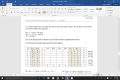# Use two JK flip-flops and a minimal two-level NAND network to implement the machine. (i). Write th

#### audreyeckman

Joined Jan 7, 2016
8
4. A state machine has one input x(t) and two-bit state (Q1(t), Q0(t)). The machine is described by the following state equations.

Q1(t + 1) = Q0(t) + x′(t) Q′1(t)

Q0(t + 1) = Q′1 (t) + x(t)Q0(t)

y(t) = Q1(t)Q0(t)

Use two JK flip-flops and a minimal two-level NAND network to implement the machine.

(i). Write the state excitation table and draw the state diagram.This is the table I have so far, Can someone please help guide me on how to fill in the JK flip flops